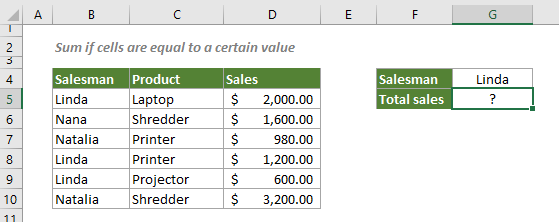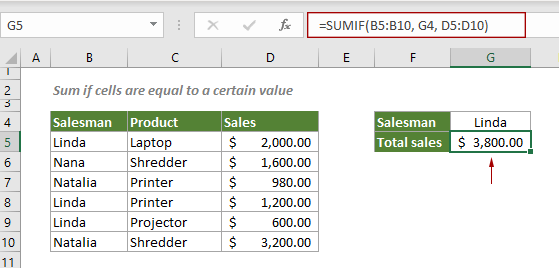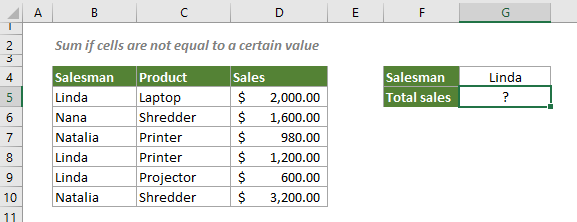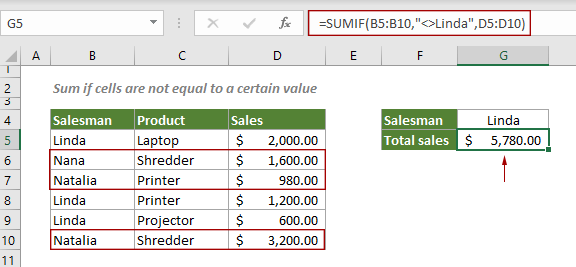## Sum if cells are equal or not equal to a certain value in Excel

This step-by-step tutorial explains two formulas to sum a range of cells that equal or not equal to a certain value based on the SUMIF function.

How to sum if cells are equal to a certain value in Excel?
How to sum if cells are not equal to a certain value in Excel?

#### How to sum if cells are equal to a certain value in Excel?

To sum the sales of the salesman “Linda” as shown in the screenshot below, follow the steps below.Generic Formulas

=SUMIF(range, criteria, sum_range)

Arguments

Range: A range of cells that need to be evaluated by the criteria;
Criteria: The criterion that defines which cells will be summed.
Sum_range: A range of cells you will sum.

How to use this formula?

1. Select a blank cell, copy or enter the formula below and press the Enter key to get the result.

=SUMIF(B5:B10,G4,D5:D10)Note: You can use an actual value in the formula like this: =SUMIF(B5:B10,"Linda", D5:D10).

Explanation of the formula

=SUMIF(B5:B10,G4,D5:D10)

If the cell values in B5:B10 equal to the salesman “Linda”, the formula will sum all corresponding sales in D5:D10.

#### How to sum if cells are not equal to a certain value in Excel?

To sum data that not equal to a certain value in Excel, the SUMIF function can also help.

As shown in the screenshot below, when the cells in the range B5:B10 are not equal to Linda, the corresponding sales in D5:D10 will be summed.Generic formula

=SUMIF(range,"<>criteria",sum_range)

Arguments

Range: A range of cells that need to be evaluated against the criteria;
Criteria: The criterion used for summing data. It can be a cell reference to a certain value or an actual value enclosed with double quotation marks;
Sum_range: A range of cells you will sum;

How to use this formula?

1. Select a blank cell, copy or enter the formula below and press the Enter key to get the result.

=SUMIF(B5:B10,"<>Linda",D5:D10)Note: Other logical operations can be used in this formula if the criterion is a number.

1) Equal to: “=number”
2) Greater than: “>number”
3) Greater than or equal to: “>=number”
4) Less than: “<number”
5) Less than or equal to: “<=number”

#### Related functions

Excel SUMIF function
The Excel SUMIF function can help to sum cells based on one criterion

#### Related formulas

Sum if cells contain an asterisk
To sum cells containing an asterisk that is regarded as a literal character not a wildcard, this tutorial explains a formula based on the SUMIF function and the tilde (~).

Sum if cells contain both x and y
This step-by-step tutorial demonstrates how to sum a range of cells if the corresponding cells containing both x and y by using the SUMIFS function.

Sum if cells contain or equal to either x or y
To sum the cells if contain or equal to either one value or another, this tutorial provides two formulas in detail to help solve the problems.

Sum if date is between two dates
This tutorial demonstrates how to sum values within a certain date range in Excel with a formula based on the SUMIFS function.

### The Best Office Productivity Tools

#### Kutools for Excel - Helps You To Stand Out From Crowd

 Popular Features: Find, Highlight or Identify Duplicates  |  Delete Blank Rows  |  Combine Columns or Cells without Losing Data  |  Round without Formula ... Super VLookup: Multiple Criteria  |  Multiple Value  |  Across Multi-Sheets  |  Fuzzy Lookup... Adv. Drop-down List: Easy Drop Down List  |  Dependent Drop Down List  |  Multi-select Drop Down List... Column Manager: Add a Specific Number of Columns  |  Move Columns  |  Toggle Visibility Status of Hidden Columns  |  Compare Columns to Select Same & Different Cells ... Featured Features: Grid Focus  |  Design View  |  Big Formula Bar  |  Workbook & Sheet Manager | Resource Library (Auto Text)  |  Date Picker  |  Combine Worksheets  |  Encrypt/Decrypt Cells  |  Send Emails by List  |  Super Filter  |  Special Filter (filter bold/italic/strikethrough...) ... Top 15 Toolsets:  12 Text Tools (Add Text, Remove Characters ...)  |  50+ Chart Types (Gantt Chart ...)  |  40+ Practical Formulas (Calculate age based on birthday ...)  |  19 Insertion Tools (Insert QR Code, Insert Picture from Path ...)  |  12 Conversion Tools (Numbers to Words, Currency Conversion ...)  |  7 Merge & Split Tools (Advanced Combine Rows, Split Excel Cells ...)  |  ... and more

Kutools for Excel Boasts Over 300 Features, Ensuring That What You Need is Just A Click Away...#### Office Tab - Enable Tabbed Reading and Editing in Microsoft Office (include Excel)

• One second to switch between dozens of open documents!
• Reduce hundreds of mouse clicks for you every day, say goodbye to mouse hand.
• Increases your productivity by 50% when viewing and editing multiple documents.
• Brings Efficient Tabs to Office (include Excel), Just Like Chrome, Edge and Firefox.